Ex 5.1

Chapter 5 Class 12 Continuity and Differentiability
Serial order wise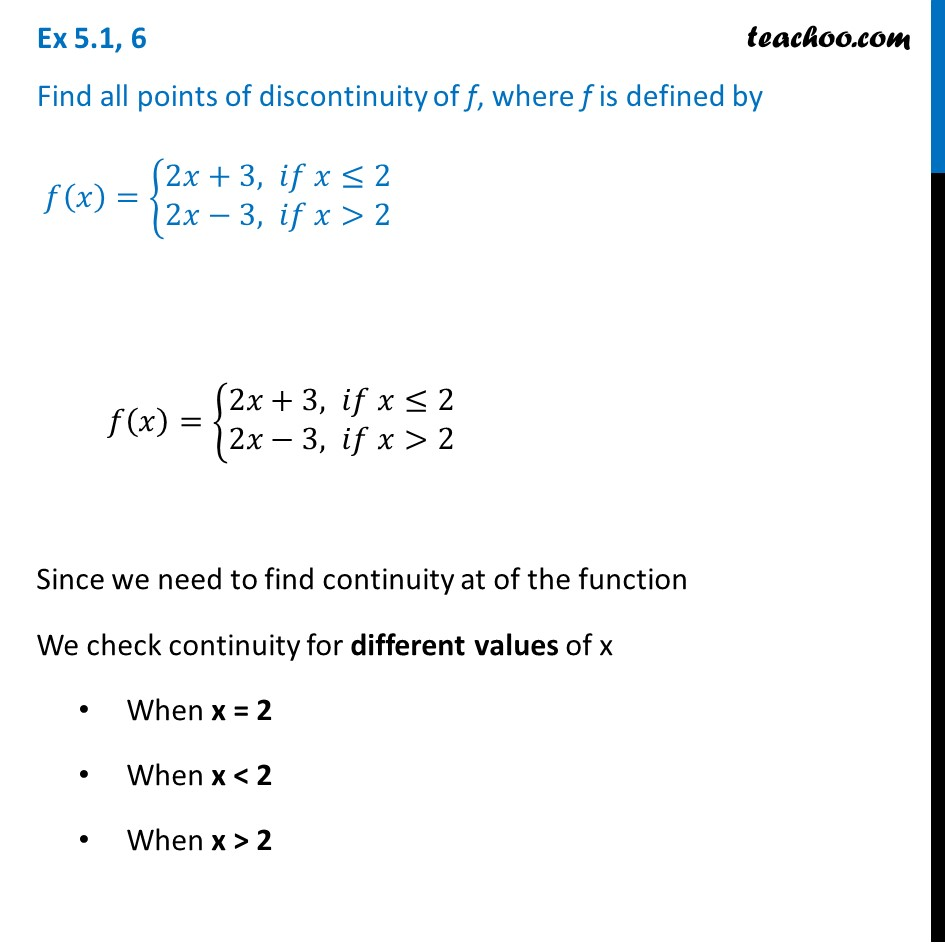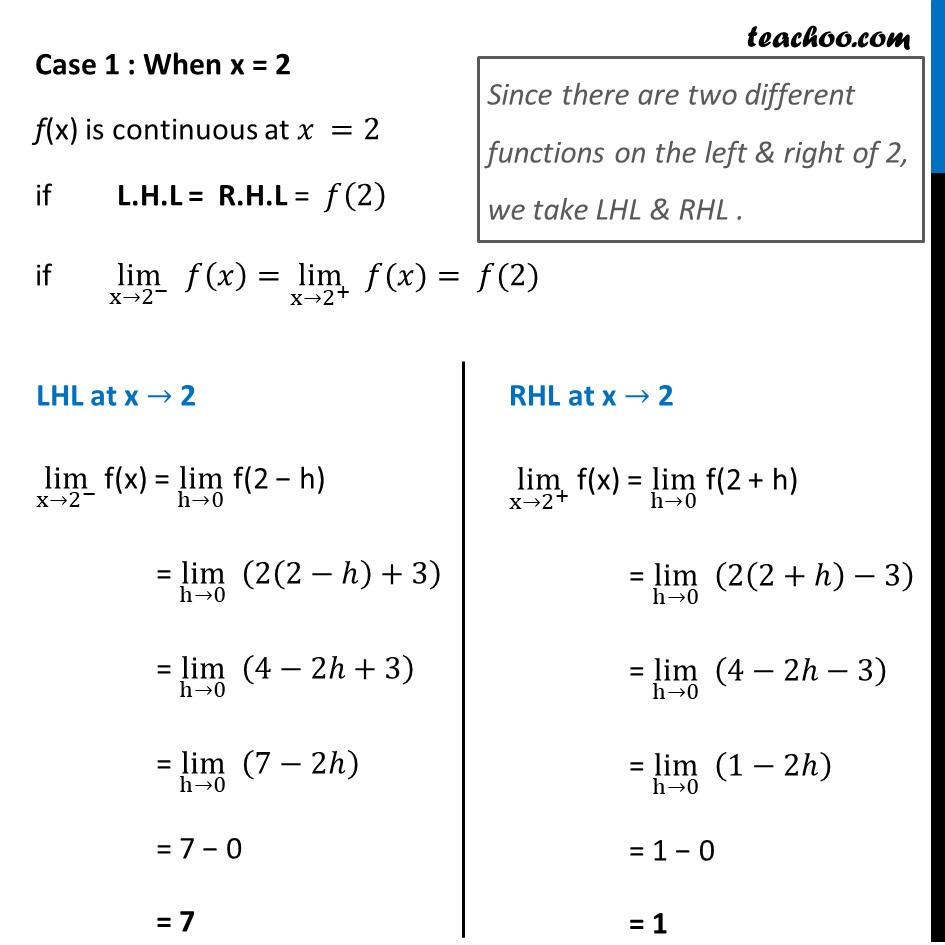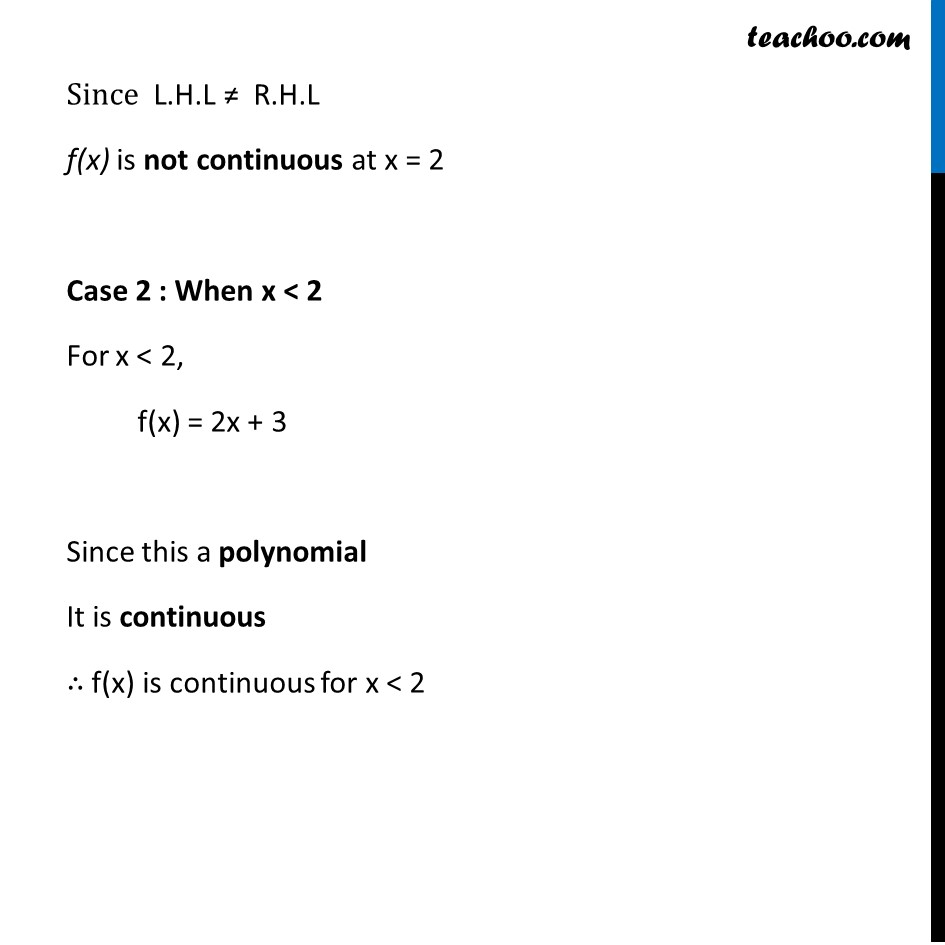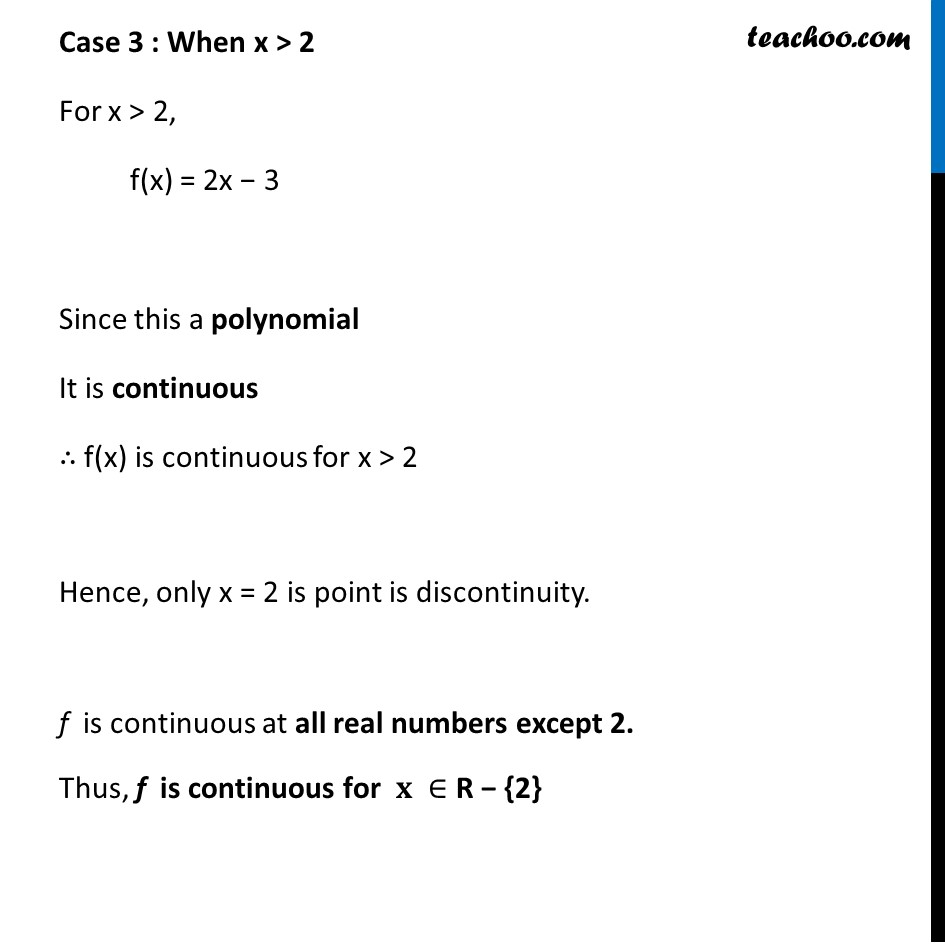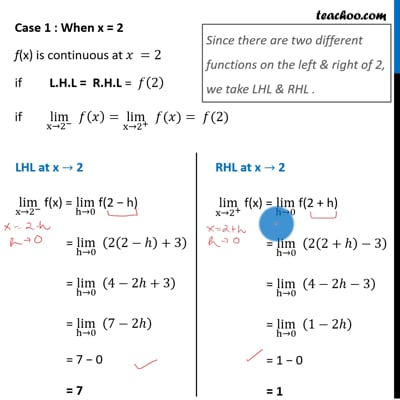This video is only available for Teachoo black users

Introducing your new favourite teacher - Teachoo Black, at only ₹83 per month

### Transcript

Ex 5.1, 6 Find all points of discontinuity of f, where f is defined by 𝑓(𝑥)={█(2𝑥+3, 𝑖𝑓 𝑥≤2@&2𝑥−3, 𝑖𝑓 𝑥>2)┤ 𝑓(𝑥)={█(2𝑥+3, 𝑖𝑓 𝑥≤2@&2𝑥−3, 𝑖𝑓 𝑥>2)┤ Since we need to find continuity at of the function We check continuity for different values of x When x = 2 When x < 2 When x > 2 Case 1 : When x = 2 f(x) is continuous at 𝑥 =2 if L.H.L = R.H.L = 𝑓(2) if lim┬(x→2^− ) 𝑓(𝑥)=lim┬(x→2^+ ) " " 𝑓(𝑥)= 𝑓(2) Since there are two different functions on the left & right of 2, we take LHL & RHL . LHL at x → 2 lim┬(x→2^− ) f(x) = lim┬(h→0) f(2 − h) = lim┬(h→0) (2(2−ℎ)+3) = lim┬(h→0) (4−2ℎ+3) = lim┬(h→0) (7−2ℎ) = 7 − 0 = 7 RHL at x → 2 lim┬(x→2^+ ) f(x) = lim┬(h→0) f(2 + h) = lim┬(h→0) (2(2+ℎ)−3) = lim┬(h→0) (4−2ℎ−3) = lim┬(h→0) (1−2ℎ) = 1 − 0 = 1 Since L.H.L ≠ R.H.L f(x) is not continuous at x = 2 Case 2 : When x < 2 For x < 2, f(x) = 2x + 3 Since this a polynomial It is continuous ∴ f(x) is continuous for x < 2 Case 3 : When x > 2 For x > 2, f(x) = 2x − 3 Since this a polynomial It is continuous ∴ f(x) is continuous for x > 2 Hence, only x = 2 is point is discontinuity. f is continuous at all real numbers except 2. Thus, f is continuous for 𝐱 ∈ R − {2}